Contents

If your browser displays incorrect colors after animation playback, look here.

Limits

The statement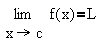can be intuitively interpreted as follows:
the number L is approached by the function values f(x) corresponding to x values that approach c.

Two examples are illustrated: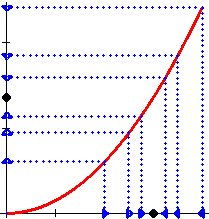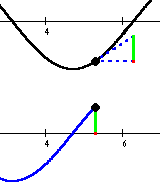Slope vs. Derivative

The top graph depicts the given function, and a triangle illustrating the rise corresponding to the run=1. As the animation advances, the triangle moves along the graph, and the rise/run values are used to create the derivative graph underneath.

Note that the positive rise/run values are indicated in green; negative values are indicated in red.

This animation can be viewed for the following functions:

Arc Length

The arc length of the graph of y=f(x) from x=a to x=b can be defined using the the length of a polygonal approximation to the graph.
The first example illustrates a sequence of polygonal approximations of a rectifiable curve, i.e., a curve with a finite arc length. In the second example, the curve has infinite length.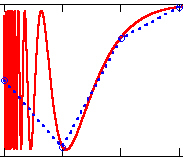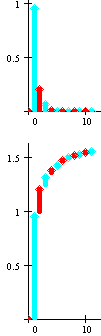Infinite Series

These animations are intended to visualize the convergence or divergence of infinite series. Two graphs are included. One of them contains the terms of the series, represented by arrows. The other one illustrates the partial sums - it uses the same arrows as the other graph, except that they are "stacked-up".

Currently, animations corresponding to the following p-series are available:

Conic Sections

We watch the cross-section of a plane and a double cone - as the plane is being rotated, different conic sections emerge. The animation includes the three-dimensional image of the cone with the plane, as well as the corresponding two-dimensional image of the plane itself. (Note that the animation file is quite large - 470K - so that it can take a while to download.)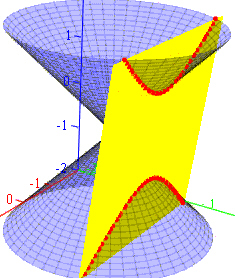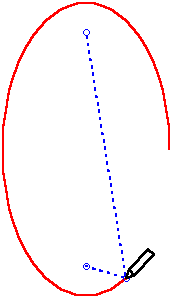Definition of Ellipse

An ellipse is a set of points in the plane, whose sum of distances from two fixed points is constant.

This definition is illustrated for two ellipses with different eccentricities

 eccentricity=4/5elongated shape eccentricity=1/5round shape

Reflective Properties

In the given conic section, several rays will be started at various points. The appropriate reflective property will only be observed when the rays originate from a focus.

 curve the rays start at a focus other point(s) Ellipsex2/9 + y2/4 = 1 ( sqrt(5), 0 ) ( 1, 1 ), ( 0, 0 ), ( 0, - sqrt(2) ), ( - 2, 0 ) Parabolay2 = x ( 0.25, 0 ) ( 1, 0 )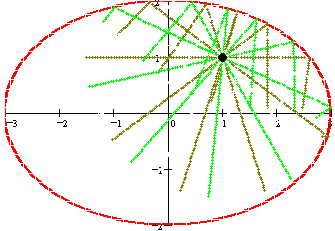Cycloids and more...

A circle of radius r is rolling on a parametric "base" curve B(t). A spoke of length R is connected to the center of the circle, and a pencil attached to the end of the spoke traces a resulting curve.
Note that the situations where the circle would get "stuck" in the base curve (e.g., due to the radius of curvature of B(t) becoming smaller than r) are not recognized. A physical interpretation of the resulting curve could be based on considering the 2-D picture to be a projection of a "thin" 3-D picture.

 base curve parameter values Line: B(t) = ( 2 pi t, 0 )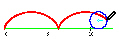r=1, R=1 (cycloid) r=1, R=2 (prolate cycloid) r=1, R=0.5 (curtate cycloid) Circle: B(t) = ( 3 cos(2 pi t), 3 sin(2 pi t) )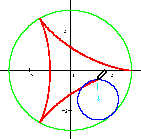r=1, R=1, inside (hypocycloid) r=1, R=0.5, inside r=1, R=1.5, inside r=1, R=1, outside (epicycloid) r=1, R=0.5, outside r=1, R=1.5, outside Parabola: B(t) = ( t, t2 - 5 )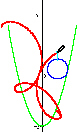r=1, R=1, inside r=0.9, R=1.5, inside r=0.5, R=1.5, inside r=1, R=1, outside r=1, R=1.5, outside r=1, R=0.5, outside

Area Inside a Parametric Curve

The area inside a parametric curve (x(t),y(t)) can be calculated by the following formulas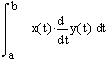or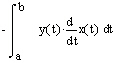if the curve is traversed counterclockwise, and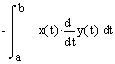or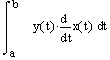if the curve is traversed clockwise.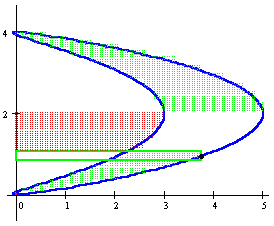We illustrate the use of these formulas for calculation of area in the two examples below. Definite integrals are approximated with the corresponding Riemann sums, and each animation frame corresponds to adding one term to the sum. The area corresponding to the current term is enclosed in a rectangle - if the term is positive, then the rectangle is green, otherwise it is red.
The partial sum of the terms included already is depicted using a dotted pattern - the dots are green within region whose area is accounted for with a factor +1; the red dots correspond to regions accounted for with a factor -1.

 Area inside the curve Formula used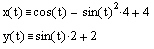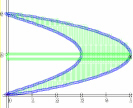counterclockwiseAVI file size: 134K (or try a shorter animation with 83K)AVI file size: 166K (or try a shorter animation with 102K)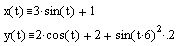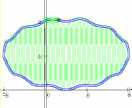clockwiseAVI file size: 75KAVI file size: 73K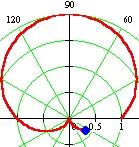Polar Curves

During the animation of each polar plot, the corresponding values of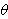and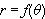will be tabulated. Animations of the following polar curves are available:

 limacons: rosecurves: spiral: circle:

 Quadric Surface Cross sections with planes parallel to Elliptic paraboloid x2 + y2 = z the xy-plane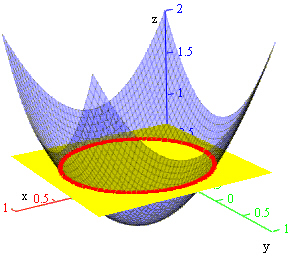the xz-plane the yz-plane Hyperbolic paraboloid x2 - y2 = z the xy-plane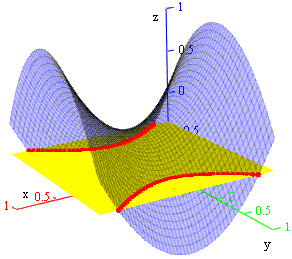the xz-plane the yz-plane